# Elements of Base R Graphic

### Basic Functions of Base R Graphics

In this blog, main objective is to introduce main and basic elements of Base R Graphics.

The blog will try to answer following questions

• How to summarizing data using scatter plot?
• How to make a scatter plot looks beautiful?
• How to change plot options?
• How to change plotting symbols?
• How to add custom labels?
• How to change colors of main title, y and y axis titles?
• How to text to data points?
• How to overlay a line to a plot?

plot : Plotting function for scatter and line charts

abline : Adding line to a plot, the line can be estimated using line or lm functions

lines : Adding line to a plot based on X and Y vectors with connecting dots

par : Setting properties of the graphic parameters /characters or symbols

legend: Legend formatting

text : Adding text to a graph or plot

points: Adding points to a graph or plot

title : Adding and formatting label to a plot

axis : Adding custom axis labels and formatting

#### Scatter Plot using plot function

Using plot function for plotting scatter plot. For athletes who participated in London Olympic, we have Height and Weight information and we could plot a scatter plot to see the relation between these two variables.

# Read data
setwd("C:/Ram/Learn R/training")
names(london)
##   "X.1"        "X"          "Name"       "Country"    "Age"
##   "Height"     "Weight"     "Sex"        "DOB"        "Place_dob"
##  "Gold"       "Silver"     "Bronze"     "Total"      "Sport"
##  "Event"      "WeightNorm" "HeightNorm" "cluster"
# Plot
plot(london$Height, london$Weight)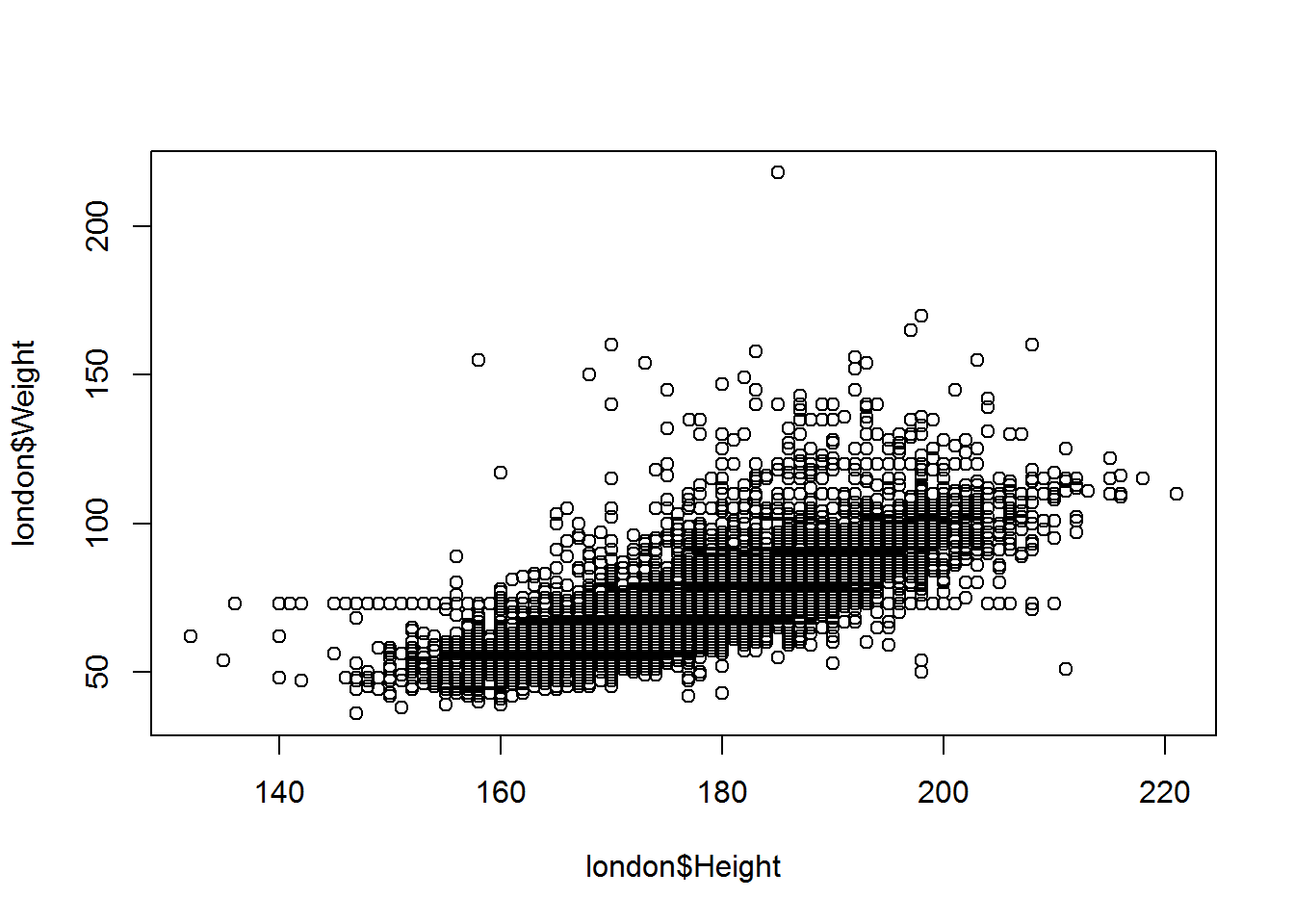Now, we need to add some labels and format the graphs to make it presentable and readable.

• main : Adding Plot title
• sub : Adding sub-title to a plot
• xlab : X Axis Title
• ylab : Y Axis Title
# Scatter Plot with Labels
plot(x=london$Height, y=london$Weight,
main="Weight Vs Height",
sub="London Olympic Athletes",
xlab="Height",
ylab="Weight"
)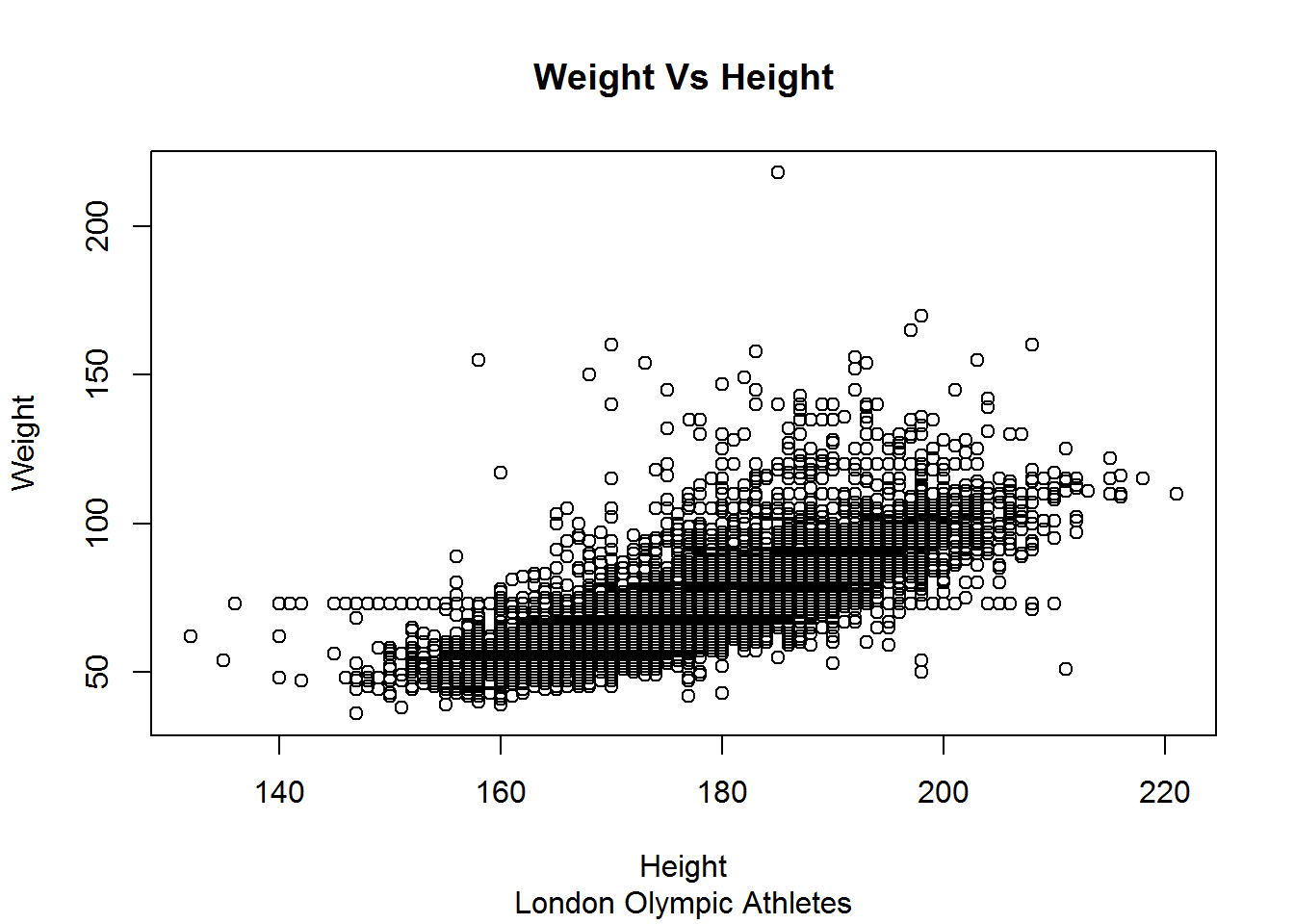Now, we can use different plotting characters to make the chart colourful and beautiful.

pch plotting characters and it takes values between 0 and 18. col change color of the plotting character

# Colorful Scatter Plot
plot(x=london$Height, y=london$Weight,
main="Weight Vs Height",
sub="London Olympic Athletes",
xlab="Height",
ylab="Weight",
pch=20,
col="blue"
)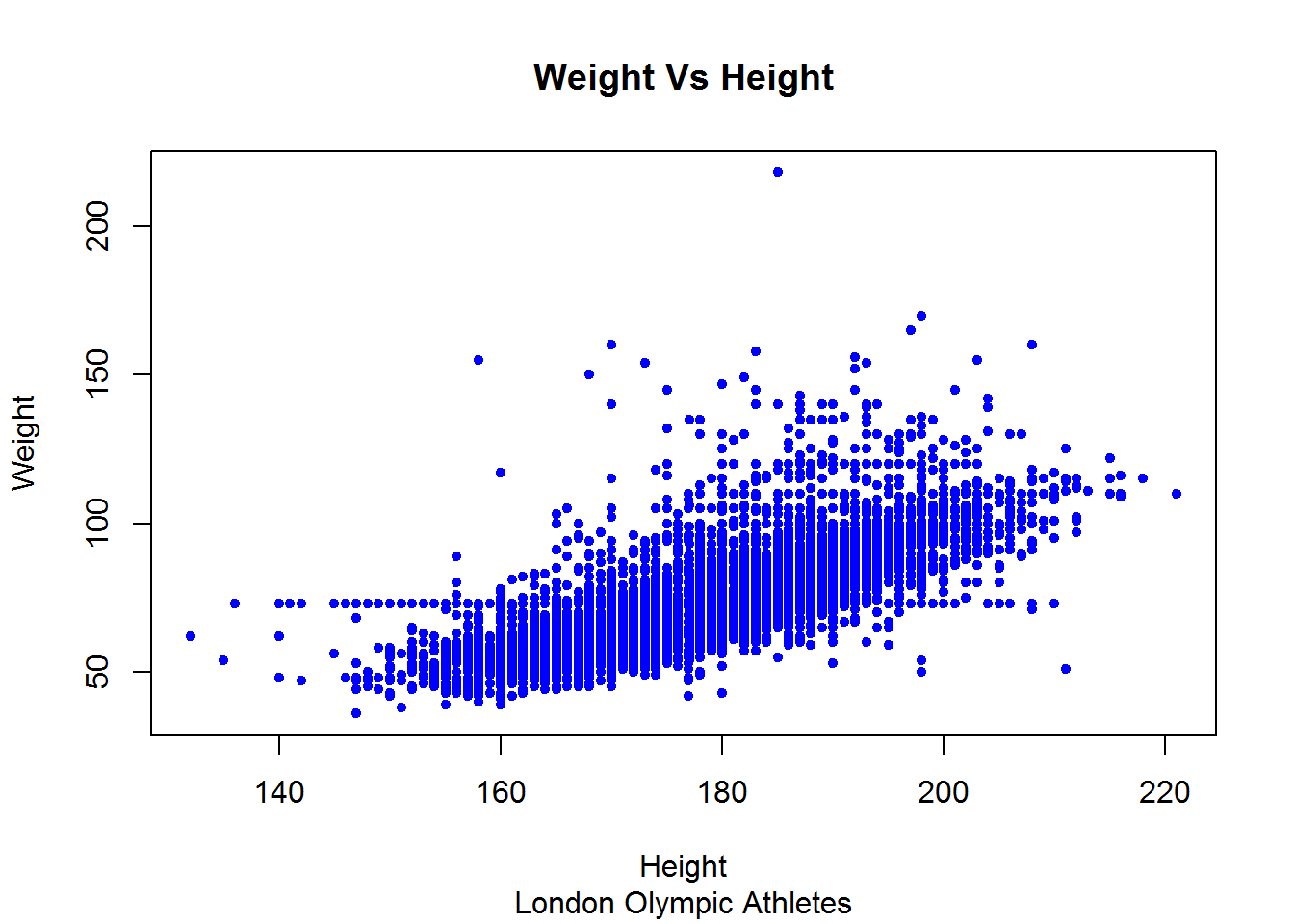Now, we may want to fit and add a line to the plot.

## add a line to plot
plot(x=london$Height, y=london$Weight,
main="Weight Vs Height",
sub="London Olympic Athletes",
xlab="Height",
ylab="Weight",
pch=20,
col="blue"
)
lm <- lm(london$Weight~london$Height)
abline(lm,
col="red",
lty=3,
lwd=3
)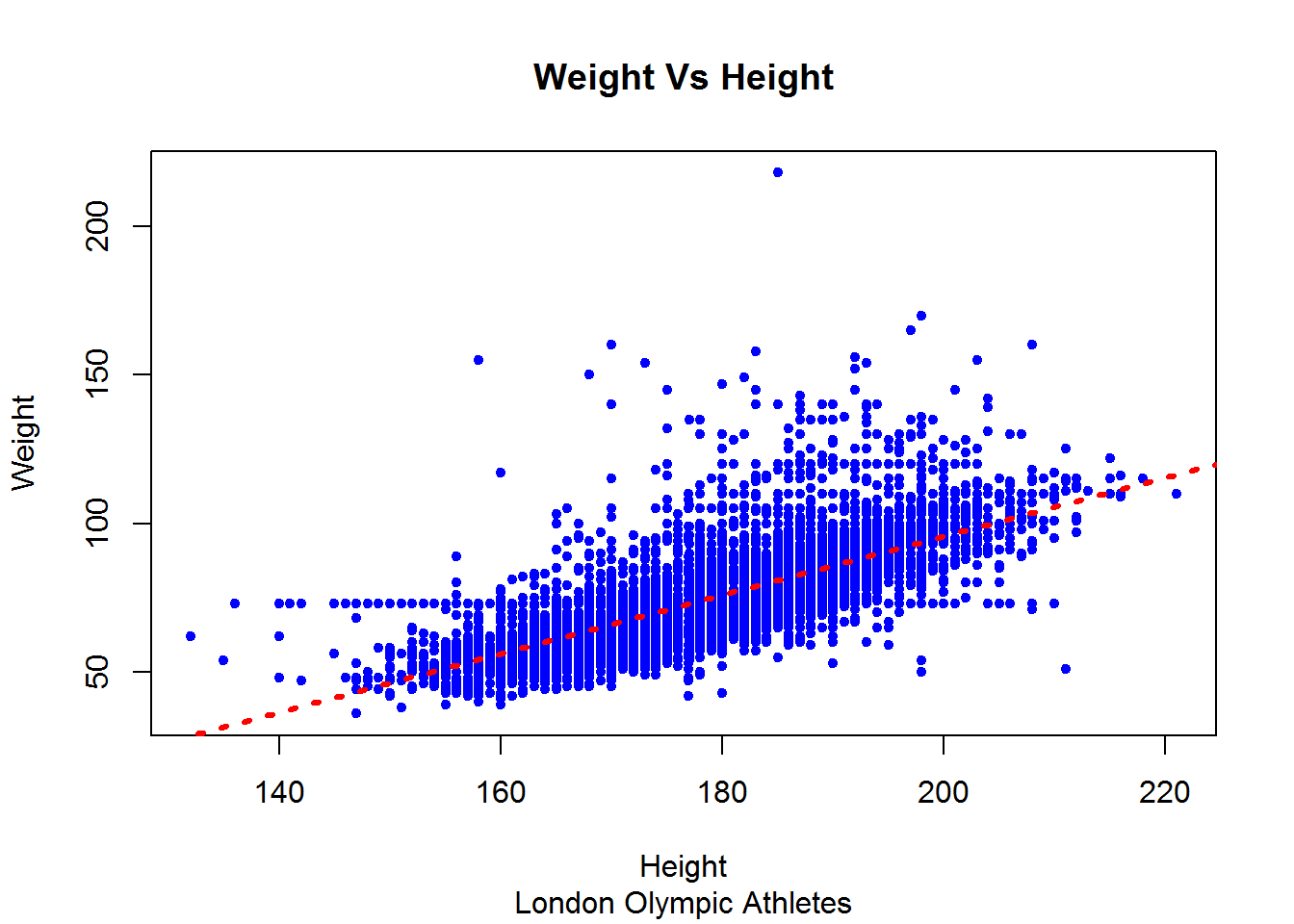Formatting axis labels and titles using par

plot.new()
par(
mfrow=c(1,2), # split window into different plotting places in this case 2 cols
bg="white", # background color of the device region
pch=15,  # Plotting character symbol
cex=0.8, # Plotting text and symbol size
col="green", # Plotting Color
col.axis="blue", # Axis color
col.lab="red", # Axis label color
col.main="orange", # title color
col.sub ="green",
font.lab=2, # 1: plain 2:bold 3:italic and 4 bold italic
lab = c(10,10,7), # c(x, y, len) - vector for tickmarks
las = 2 # 0: parallel 1: Horizonal 2 : Perpendicular 3: Always vertical
)

# Colorful Scatter Plot : Male
Male <- london$Sex=="M" plot(x=london$Height[Male],
y=london$Weight[Male], main="Weight Vs Height:Male", sub="London Olympic Athletes", xlab="Height", ylab="Weight" ) # Estimate line line.est <- line(x=london$Height[Male],
y=london$Weight[Male] ) abline(line.est, col="red", lty=1, lwd=3 ) # Colorful Scatter Plot : Female Female <- london$Sex=="F"
plot(x=london$Height[Female], y=london$Weight[Female],
main="Weight Vs Height: Female",
sub="London Olympic Athletes",
xlab="Height",
ylab="Weight"
)
fline.est <- line(x=london$Height[Female], y=london$Weight[Female]
)
abline(fline.est,
col="red",
lty=1,
lwd=3
)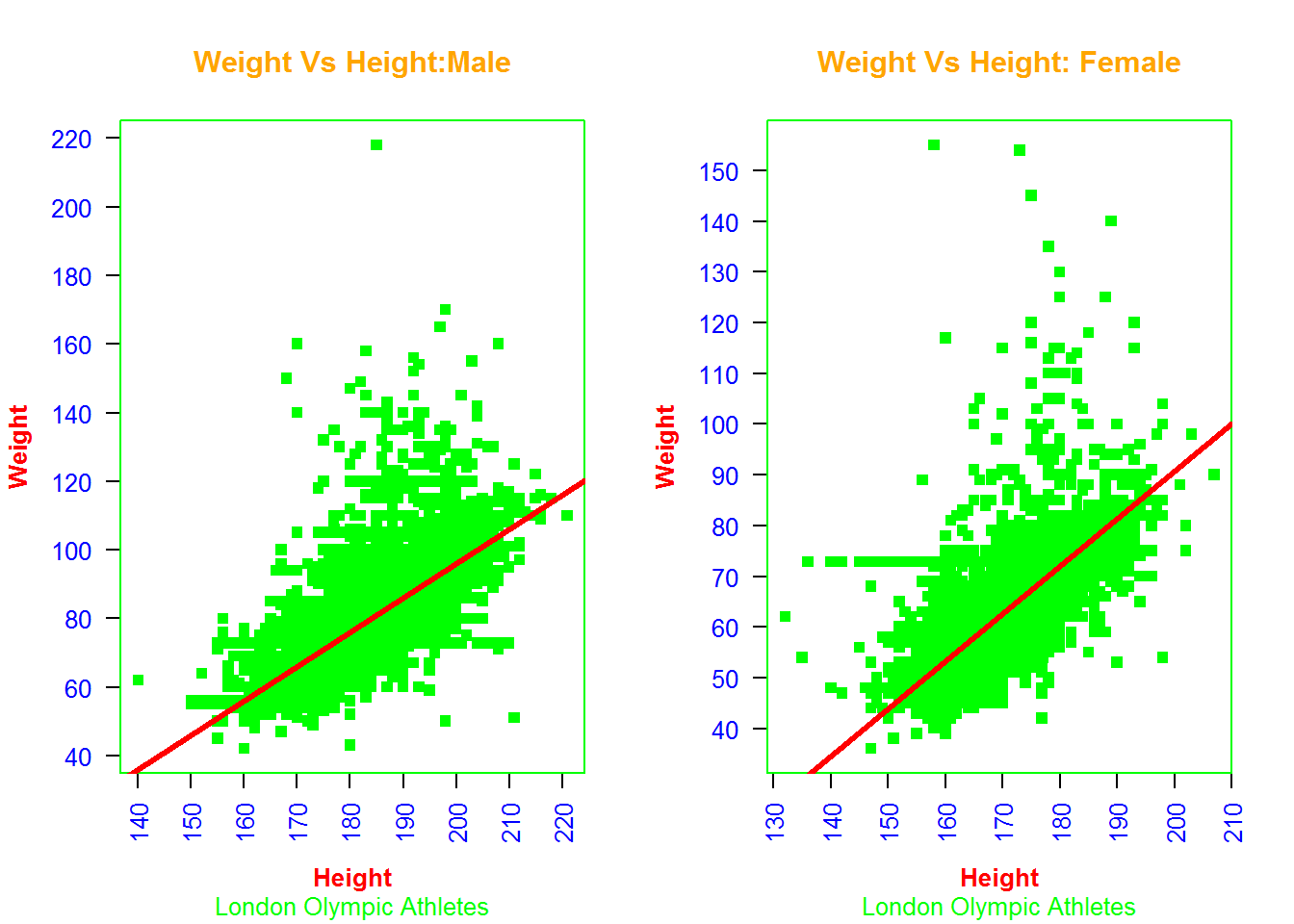We can create a factor variable for Height and plot average/mean of the weights.

axis is used for giving labels. xaxt option in plot is used for suppressing default label. axis function computes automatically the tick marks position, but we can reset manually using at option in this function

title is shown below for changing formatting of the title.

text is used for adding text labels to the points on the graph.

par(mfrow=c(1,1))
# Create Groups/factors
Height.Grp <- cut(london$Height, breaks=c(160,170,180,190,300),labels=c("Low-170","170-180","180-190","190-High")) # Summarize weights by Height Factors mean.weight <- aggregate(london$Weight,by=list(Height.Grp),mean)
names(mean.weight) <-c("HeightGroup","Avg.Weight")

plot( mean.weight$Avg.Weight, col="red", xaxt = 'n', xlab="Height Level", ylab="Mean Weight", pch=20 ) # add and format axis label axis(1, at=1:4, labels=mean.weight$HeightGroup
)
# Format title
title(main="Height and Weight",
font.main=15,
col.main="blue",
cex.main=2)
# add text to points on plot
label.position <- mean.weight$Avg.Weight + c(1,2,2,-2) text(1:4, y=label.position, labels=round(mean.weight$Avg.Weight,1),
col="orange",
cex=0.8
)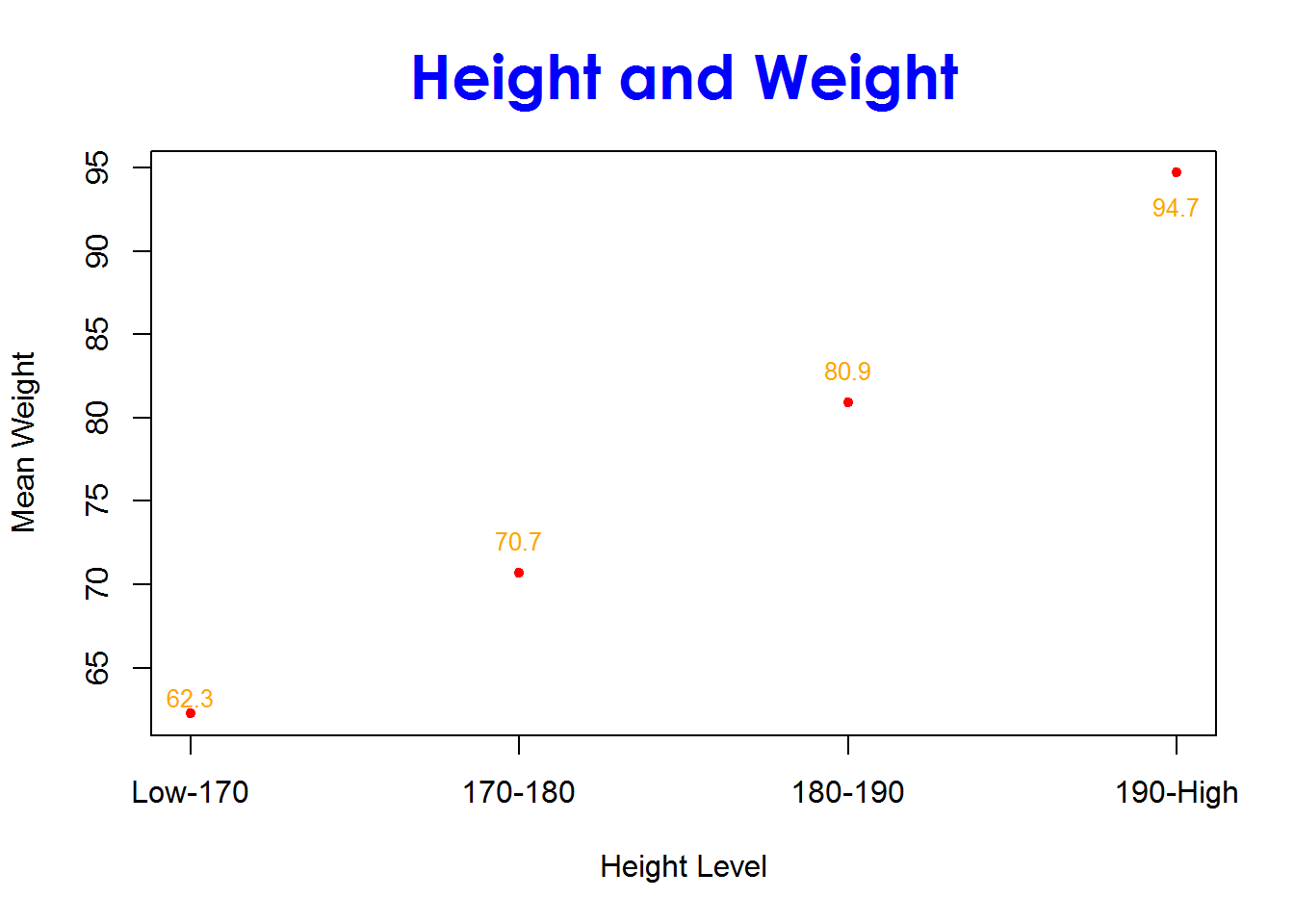So far we have discussed on Base R graphics mainly for scatter plot. In next blog ( http://dni-institute.in/blogs/line-chart-using-base-r/) we are going to use plot function for line chart and also use additional functions for making the visualization powerful.

Reference

http://www.dummies.com/how-to/content/how-to-change-plot-options-in-r.html